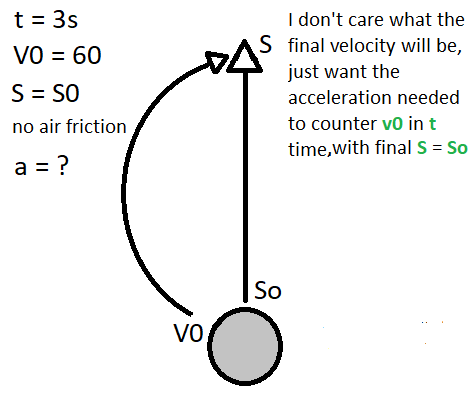# How do I calculate acceleration for a curve effect?

On my golf game I use some physics formulas like projectile motion and friction motion to calculate how much distance the ball will travel, and they all seem to work fine. Then I tried to do a curve effect option, and I failed miserably on the calculations, so the ball always fell on the ground too much to the left or to the right. I don’t want this because I want the players to have a precise, consistent experience.

I wanted to know what formula could be used to know how much acceleration (a) an object needs to counter an initial velocity (Vo) in a certain amount of time (t), where the final position (S) will be the same as the initial position (So). There is no air friction or wind.

Here is an example of what it should be like:It should be something like a = (2 * V0)/T to create that nice symmetric curve effect

1 Like

Thanks a lot! It’s working fine now. I already tried that solution before but it didn’t work for me. Then, I went into looking the S=So+Vot+at*t/2 formula again to check how I could get acceleration and reached the same formula you used again. And finally, I have found what was wrong in my code: I didn’t use :GetMass() on the ball to multiply the result.
Here is the result (aimed right at the hole flag direction):

1 Like